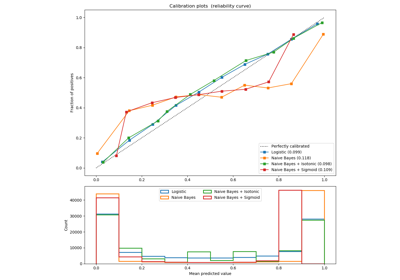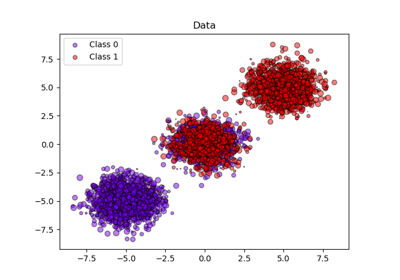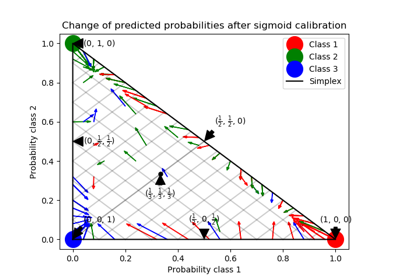# sklearn.calibration.CalibratedClassifierCV¶

class sklearn.calibration.CalibratedClassifierCV(base_estimator=None, method='sigmoid', cv=None)[source]

Probability calibration with isotonic regression or sigmoid.

See glossary entry for cross-validation estimator.

With this class, the base_estimator is fit on the train set of the cross-validation generator and the test set is used for calibration. The probabilities for each of the folds are then averaged for prediction. In case that cv=”prefit” is passed to __init__, it is assumed that base_estimator has been fitted already and all data is used for calibration. Note that data for fitting the classifier and for calibrating it must be disjoint.

Read more in the User Guide.

Parameters
base_estimatorinstance BaseEstimator

The classifier whose output decision function needs to be calibrated to offer more accurate predict_proba outputs. If cv=prefit, the classifier must have been fit already on data.

method‘sigmoid’ or ‘isotonic’

The method to use for calibration. Can be ‘sigmoid’ which corresponds to Platt’s method or ‘isotonic’ which is a non-parametric approach. It is not advised to use isotonic calibration with too few calibration samples (<<1000) since it tends to overfit. Use sigmoids (Platt’s calibration) in this case.

cvinteger, cross-validation generator, iterable or “prefit”, optional

Determines the cross-validation splitting strategy. Possible inputs for cv are:

• None, to use the default 5-fold cross-validation,

• integer, to specify the number of folds.

• An iterable yielding (train, test) splits as arrays of indices.

For integer/None inputs, if y is binary or multiclass, sklearn.model_selection.StratifiedKFold is used. If y is neither binary nor multiclass, sklearn.model_selection.KFold is used.

Refer User Guide for the various cross-validation strategies that can be used here.

If “prefit” is passed, it is assumed that base_estimator has been fitted already and all data is used for calibration.

Changed in version 0.22: cv default value if None changed from 3-fold to 5-fold.

Attributes
classes_array, shape (n_classes)

The class labels.

calibrated_classifiers_list (len() equal to cv or 1 if cv == “prefit”)

The list of calibrated classifiers, one for each crossvalidation fold, which has been fitted on all but the validation fold and calibrated on the validation fold.

References

R57cf438d7060-1

Obtaining calibrated probability estimates from decision trees and naive Bayesian classifiers, B. Zadrozny & C. Elkan, ICML 2001

R57cf438d7060-2

Transforming Classifier Scores into Accurate Multiclass Probability Estimates, B. Zadrozny & C. Elkan, (KDD 2002)

R57cf438d7060-3

Probabilistic Outputs for Support Vector Machines and Comparisons to Regularized Likelihood Methods, J. Platt, (1999)

R57cf438d7060-4

Predicting Good Probabilities with Supervised Learning, A. Niculescu-Mizil & R. Caruana, ICML 2005

Methods

 fit(self, X, y[, sample_weight]) Fit the calibrated model get_params(self[, deep]) Get parameters for this estimator. predict(self, X) Predict the target of new samples. predict_proba(self, X) Posterior probabilities of classification score(self, X, y[, sample_weight]) Return the mean accuracy on the given test data and labels. set_params(self, \*\*params) Set the parameters of this estimator.
__init__(self, base_estimator=None, method='sigmoid', cv=None)[source]

Initialize self. See help(type(self)) for accurate signature.

fit(self, X, y, sample_weight=None)[source]

Fit the calibrated model

Parameters
Xarray-like, shape (n_samples, n_features)

Training data.

yarray-like, shape (n_samples,)

Target values.

sample_weightarray-like of shape (n_samples,), default=None

Sample weights. If None, then samples are equally weighted.

Returns
selfobject

Returns an instance of self.

get_params(self, deep=True)[source]

Get parameters for this estimator.

Parameters
deepbool, default=True

If True, will return the parameters for this estimator and contained subobjects that are estimators.

Returns
paramsmapping of string to any

Parameter names mapped to their values.

predict(self, X)[source]

Predict the target of new samples. Can be different from the prediction of the uncalibrated classifier.

Parameters
Xarray-like, shape (n_samples, n_features)

The samples.

Returns
Carray, shape (n_samples,)

The predicted class.

predict_proba(self, X)[source]

Posterior probabilities of classification

This function returns posterior probabilities of classification according to each class on an array of test vectors X.

Parameters
Xarray-like, shape (n_samples, n_features)

The samples.

Returns
Carray, shape (n_samples, n_classes)

The predicted probas.

score(self, X, y, sample_weight=None)[source]

Return the mean accuracy on the given test data and labels.

In multi-label classification, this is the subset accuracy which is a harsh metric since you require for each sample that each label set be correctly predicted.

Parameters
Xarray-like of shape (n_samples, n_features)

Test samples.

yarray-like of shape (n_samples,) or (n_samples, n_outputs)

True labels for X.

sample_weightarray-like of shape (n_samples,), default=None

Sample weights.

Returns
scorefloat

Mean accuracy of self.predict(X) wrt. y.

set_params(self, **params)[source]

Set the parameters of this estimator.

The method works on simple estimators as well as on nested objects (such as pipelines). The latter have parameters of the form <component>__<parameter> so that it’s possible to update each component of a nested object.

Parameters
**paramsdict

Estimator parameters.

Returns
selfobject

Estimator instance.

## Examples using sklearn.calibration.CalibratedClassifierCV¶